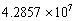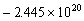Transforming Numerical Methods Education for the STEM Undergraduate MOOC | MOBILE | VIDEOS | BLOG | YOUTUBE | TWITTER | COMMENTS | ANALYTICS | ABOUT | CONTACT | COURSE WEBSITES | BOOKS | MATH FOR COLLEGE
 MULTIPLE CHOICE TEST GAUSSIAN ELIMINATION SIMULTANEOUS LINEAR EQNS

Q1. The goal of forward elimination steps in the Naïve Gauss elimination method is to reduce the coefficient matrix to a (an) _____________  matrix.

diagonal

identity

lower triangular

upper triangular

Q2. Division by zero during forward elimination steps in Naïve Gaussian elimination of the set of equations [A][X]=[C] implies the coefficient matrix [A] is

invertible

nonsingular

not determinable to be singular or nonsingular

singular

Q3. Using a computer with four significant digits with chopping, Naïve Gauss elimination solution tois

x1 = 26.66; x2 = 1.051

x1 = 8.769; x2 = 1.051

x1 = 8.800; x2 = 1.000

x1 = 8.771; x2 = 1.052

Q4. Using a computer with four significant digits with chopping, Gauss elimination with partial pivoting solution tois

x1 = 26.66; x2 = 1.051

x1 = 8.769; x2 = 1.051

x1 = 8.800; x2 = 1.000

x1 = 8.771; x2 = 1.052

Q5. At the end of forward elimination steps of Naïve Gauss Elimination method on the following equationsthe resulting equations in the matrix form are given byThe determinant of the original coefficient matrix is

0.00Q6. The following data is given for the velocity of the rocket as a function of time.  To find the velocity at t=21 s, you are asked to use a quadratic polynomial, v(t)=at2+bt+c to approximate the velocity profile.

 t (s) 0 14 15 20 30 35 v(t) m/s 0 227.04 362.78 517.35 602.97 901.67

The correct set of equations that will find a, b and c areMultiple choice questions on other topics